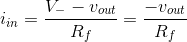# Transimpedance Amplifier

## Working principle

A transimpedance amplifier is a current-to-voltage converter, which outputs a voltage equal to the negative product of the input current and the feedback resistor (Rf): vout = -iinRf.

### Formula derivation

Because of virtual ground and infinite impedance of the op-amp’s input terminals, all of the input current flows through Rf, which means that the output voltage is proportional to the resistor voltage and, thus, the input current:The gain of the amplifer is set by Rf and, because the amplifier is in an inverting configuration, has a negative value.

Current-to-voltage converters are used with sensors that have a current response that is more linear than the voltage response, such as a photodiode – to accurately sense the amount of light in an environment.

### Experiment

• Vary the input current amplitude and frequency and verify that the output voltage directly follows the input.